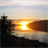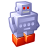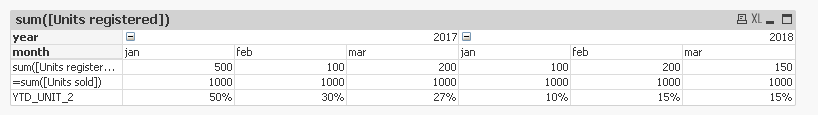# QlikView App Dev

Discussion Board for collaboration related to QlikView App Development.

Announcements
Skip the ticket, Chat with Qlik Support instead for instant assistance.
cancel
Showing results for
Did you mean:Creator

## Calculating all months in a year per month

I'm not sure how to explain this in words, therefore, i've added a table.

How can i calculate the conversation rate with Qlikview? 🙂

 jan-18 feb-18 mrt-18 apr-18 Units registered 100 200 150 200 Units sold 1000 1000 1000 1000 Conversion 10% 15% 15% 16%

Conversion:
Jan-18: Sum(Units Registered JAN)\(Units Sold JAN)
Feb-18: Sum(Units Registered JAN + FEB )\(Units Sold JAN + FEB)
Mar-18:Feb-18: Sum(Units Registered JAN + FEB + MAR )\(Units Sold JAN + FEB + MAR)
Apr-18:Feb-18: Sum(Units Registered JAN + FEB + MAR + APR )\(Units Sold JAN + FEB+MAR + APR)

And this for multiple years. Every year follows the same logic

1 Solution

Accepted SolutionsPartner - Creator

Hi,

You can try this.

divide

jan Units_registered/jan units sold for (Jan Conversion) in the expression.(Jan)

Feb Units_registered/Feb units sold (Feb Conversion) and so on. (jan+feb). (attached qvw)

Regards,

Shreya

4 RepliesPartner - Creator

Hi,

You can try this.

divide

jan Units_registered/jan units sold for (Jan Conversion) in the expression.(Jan)

Feb Units_registered/Feb units sold (Feb Conversion) and so on. (jan+feb). (attached qvw)

Regards,

ShreyaContributor III

Hello,
To do it with an expression you could perform the following steps:

1º I have loaded the indicated data into a table, separating the months and the years as in the following example:

year, month, Units registered, Units sold
2017, jan, 500, 1000
2017, feb, 100, 1000
2017, mar, 200, 1000
2018, jan, 100, 1000
2018, feb, 200, 1000
2018, mar, 150, 1000];

2º In a pivot table you have to have the years and months as columns and the expressions as rows (as you indicate in your example).3º The expressions would be the following:

1º expression: sum([Units registered])

2º expression: sum([Units sold])

3º expression (Conversion):

if(month = 'jan', sum([Units registered])/sum([Units sold]),
if(month= 'feb', (Before(sum([Units registered]))+sum([Units registered]))/(Before(sum([Units sold]))+sum([Units sold])),
if(month= 'mar', (Before(sum([Units registered]), 2)+Before(sum([Units registered]))+sum([Units registered]))/(Before(sum([Units sold]), 2)+Before(sum([Units sold]))+sum([Units sold])), 0)))

For the Conversion I give you an example with 3 months, it would be to extend the "IF" until 12 months. This expression would be valid for all the years you had.

GreetingsContributor III

I forgot to tell you that in the expression of Conversion for each month, you have to add the previous month with the expression of "BEFORE".

For example for April it is:

if(mes= 'apr', (Before(sum([Units registered]), 3)+Before(sum([Units registered]), 2)+Before(sum([Units registered]))+sum([Units registered]))/(Before(sum([Units sold]), 3)+Before(sum([Units sold]), 2)+Before(sum([Units sold]))+sum([Units sold])), ......next expression.

Greetings,Creator
Author
Thanks, this seems to work!

I also created a rolling calendar in Excel, and attached it to my normal calendar, that also did the trick.

Jan 2017 - Jan 2017
Feb 2017 - Jan 2017
Feb 2017 - Feb 2017
Mar 2017 - Jan 2017
Mar 2017 - Feb 2017
Mar 2017 - Mar 2017

Etc.Community Browser